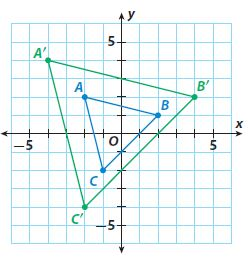Homework Explained - Math Practice 101Dear guest, you are not a registered member. As a guest, you only have read-only access to our books, tests and other practice materials.

As a registered member you can:

Registration is free and doesn't require any type of payment information. Click here to Register.
Go to page:
Chapter 10:Transformations and Similarity; Lesson 1: Properties of Dilations

### Guided Practice

Use triangles ABC and A′B′C ′ for 1–5.• Question 1

For each pair of corresponding vertices, find the ratio of the x-coordinates and the ratio of the y-coordinates.

ratio of x-coordinates = _______

ratio of y-coordinates = _______

•  ratio of x-coordinates = ratio of y-coordinates =
• Question 2

I know that triangle A′B′C ′ is a dilation of triangle ABC because the ratios of the corresponding x-coordinates are _______ and the ratios of the corresponding y-coordinates are _______.

• Type below:
• Question 3

The ratio of the lengths of the corresponding sides of triangle A′B′C ′ and triangle ABC equals _______.

• Question 4

The corresponding angles of triangle ABC and triangle A′B′C ′ are _______.

• Type below:
• Question 5

The scale factor of the dilation is _______.

### ESSENTIAL QUESTION CHECK-IN

• Question 6

How can you find the scale factor of a dilation?

• Type below:

Yes, email page to my online tutor. (if you didn't add a tutor yet, you can add one here)